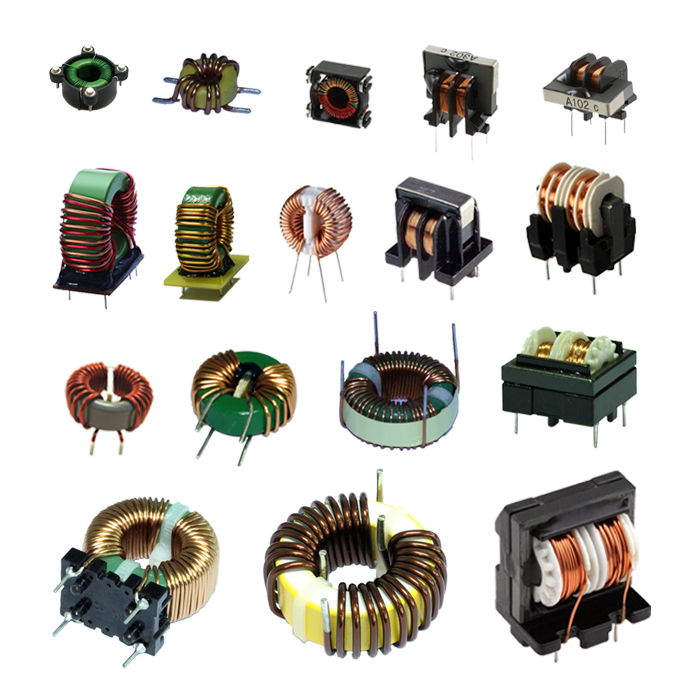# Introduction of the function of inductors

##### author: MagTop
06/04/2022Magnetic antenna coils in radios, high-frequency chokes in simple semiconductor radios, oscillating coils in superheterodyne radios, etc., are inductors.
Inductor commonly known as inductance coil or short coil, is also a common electronic components; But its use in circuits is much less than that of resistors and capacitors. So what exactly is inductance? What role can it play in the circuit?

1)
R
esistance / 阻流: the self-induced electromotive force in the coil is
Always opposed to the current change in the coil. It can be divided into high frequency choke coil and low frequency choke coil.

2)Tuning and frequency selection/调谐与选频: LC tuning circuit can be composed ofinductive coil and capacitor in parallel. That is, the natural  Oscillation frequency of the circuit f0 is equal to the frequency f of the non-AC signal, so the inductive reactance and capacitive reactance of thecircuit are equal, so the electromagnetic energy oscillates back and forth between the inductor and capacitor, which is the resonance phenomenonof the LC circuit. During resonance, because the inductive reactance and capacitive reactance of the circuit are equivalent to reverse, the total inductive reactance of the circuit is the minimum and the current is the maximum (referring to the AC signal f= F0), so the LC resonant circuit has the function of selecting frequency and can select the AC signal of a certain frequency F.

3)Choke/扼流: in low frequency circuit used to prevent low frequency ALTERNATING current; Pulsating DC to pure DC circuit; It is usually used in the middle of two filter capacitors at the output end of the rectifier circuit, and the choke and capacitor constitute the filter circuit. In the high frequency circuit: is to prevent the high frequency current flow to the low frequency end, in the old regenerative radio in the high frequency choke is applied.
4)Filtering/滤波: it is also to prevent the pulsating DC current after rectification from flowing to the pure DC routing choke (in order to simplify the circuit and reduce the cost, the choke is replaced by a pure resistor). Two capacitors (electrolytic capacitors) constitute the sending filter circuit.The pure DC is obtained by using the capacitor charge-discharge function and choke ring to pass DC and block ac characteristics to complete smooth DC.

5)shock/震荡: rectification is to turn alternating current into direct current,so the shock is the reverse process of turning direct current into alternating current, so the circuit to complete this process is called
"oscillator". Oscillator waveform: there are rotational wave, sawtooth wave,trapezoidal wave, square wave, rectangular wave, peak wave.  Frequencies range from a few Hertz to tens of G Hertz. It is widely used in the field of wire and radio.